Courses

# C: Loops Computer Science Engineering (CSE) Notes | EduRev

## Computer Science Engineering (CSE) : C: Loops Computer Science Engineering (CSE) Notes | EduRev

The document C: Loops Computer Science Engineering (CSE) Notes | EduRev is a part of the Computer Science Engineering (CSE) Course GATE Computer Science Engineering(CSE) 2022 Mock Test Series.
All you need of Computer Science Engineering (CSE) at this link: Computer Science Engineering (CSE)

You may encounter situations, when a block of code needs to be executed several number of times. In general, statements are executed sequentially: The first statement in a function is executed first, followed by the second, and so on.

Programming languages provide various control structures that allow for more complicated execution paths.

A loop statement allows us to execute a statement or group of statements multiple times. Given below is the general form of a loop statement in most of the programming languages −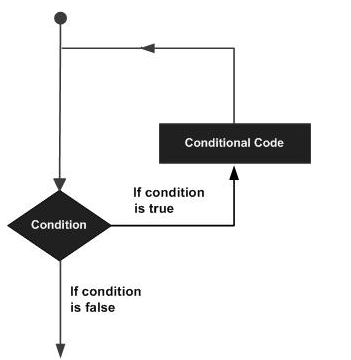C programming language provides the following types of loops to handle looping requirements.

 S.N. Loop Type & Description 1 while loopRepeats a statement or group of statements while a given condition is true. It tests the condition before executing the loop body. 2 for loopExecutes a sequence of statements multiple times and abbreviates the code that manages the loop variable. 3 do... while loop doIt is more like a while statement, except that it tests the condition at the end of the loop body. 4 nested loopsYou can use one or more loops inside any other while, for, or do..while loop.

Loop Control Statements

Loop control statements change execution from its normal sequence. When execution leaves a scope, all automatic objects that were created in that scope are destroyed.

C supports the following control statements.

 S.N. Control Statement & Description 1 break statment Terminates the loop or switch statement and transfers execution to the statement immediately following the loop or switch. 2 continue statmentCauses the loop to skip the remainder of its body and immediately retest its condition prior to reiterating. 3 goto statmentTransfers control to the labeled statement.

## The Infinite Loop

A loop becomes an infinite loop if a condition never becomes false. The for loop is traditionally used for this purpose. Since none of the three expressions that form the 'for' loop are required, you can make an endless loop by leaving the conditional expression empty.

`#include <stdio.h> int main () {   for( ; ; ) {      printf("This loop will run forever.");   }   return 0;}`

When the conditional expression is absent, it is assumed to be true. You may have an initialization and increment expression, but C programmers more commonly use the for(;;) construct to signify an infinite loop.

## while loop

while loop in C programming repeatedly executes a target statement as long as a given condition is true.

## Syntax

The syntax of a while loop in C programming language is −

`while(condition) {   statement(s);}`

Here, statement(s) may be a single statement or a block of statements. The condition may be any expression, and true is any nonzero value. The loop iterates while the condition is true.

When the condition becomes false, the program control passes to the line immediately following the loop.

## Flow Diagram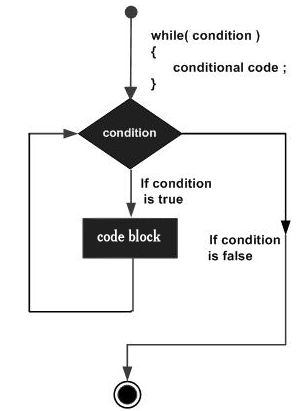Here, the key point to note is that a while loop might not execute at all. When the condition is tested and the result is false, the loop body will be skipped and the first statement after the while loop will be executed.

## Example

`#include <stdio.h> int main () {   /* local variable definition */   int a = 10;   /* while loop execution */   while( a < 20 ) {      printf("value of a: %d", a);      a++;   }    return 0;}`

When the above code is compiled and executed, it produces the following result −

`value of a: 10value of a: 11value of a: 12value of a: 13value of a: 14value of a: 15value of a: 16value of a: 17value of a: 18value of a: 19`

## for loop

for loop is a repetition control structure that allows you to efficiently write a loop that needs to execute a specific number of times.

## Syntax

The syntax of a for loop in C programming language is −

`for ( init; condition; increment ) {   statement(s);}`

Here is the flow of control in a 'for' loop −

• The init step is executed first, and only once. This step allows you to declare and initialize any loop control variables. You are not required to put a statement here, as long as a semicolon appears.

• Next, the condition is evaluated. If it is true, the body of the loop is executed. If it is false, the body of the loop does not execute and the flow of control jumps to the next statement just after the 'for' loop.

• After the body of the 'for' loop executes, the flow of control jumps back up to the increment statement. This statement allows you to update any loop control variables. This statement can be left blank, as long as a semicolon appears after the condition.

• The condition is now evaluated again. If it is true, the loop executes and the process repeats itself (body of loop, then increment step, and then again condition). After the condition becomes false, the 'for' loop terminates.

## Flow Diagram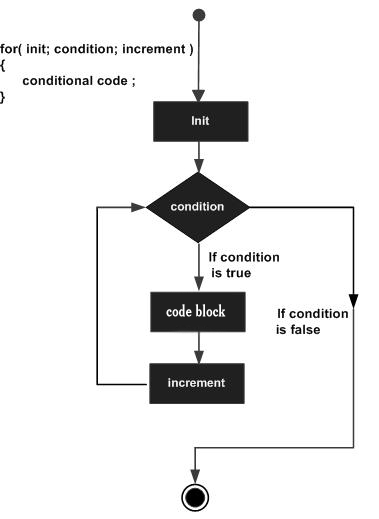## Example

`#include <stdio.h> int main () {   int a;	   /* for loop execution */   for( a = 10; a < 20; a = a + 1 ){      printf("value of a: %d", a);   }    return 0;}`

When the above code is compiled and executed, it produces the following result −

`value of a: 10value of a: 11value of a: 12value of a: 13value of a: 14value of a: 15value of a: 16value of a: 17value of a: 18value of a: 19`

## do...while loop

Unlike for and while loops, which test the loop condition at the top of the loop, the do...while loop in C programming checks its condition at the bottom of the loop.

do...while loop is similar to a while loop, except the fact that it is guaranteed to execute at least one time.

## Syntax

The syntax of a do...while loop in C programming language is −

`do {   statement(s);} while( condition );`

Notice that the conditional expression appears at the end of the loop, so the statement(s) in the loop executes once before the condition is tested.

If the condition is true, the flow of control jumps back up to do, and the statement(s) in the loop executes again. This process repeats until the given condition becomes false.

## Flow Diagram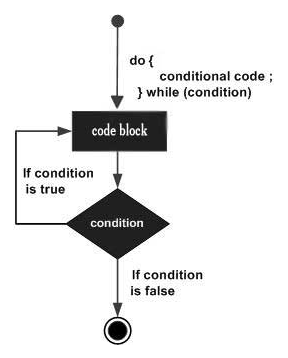## Example

`#include <stdio.h> int main () {   /* local variable definition */   int a = 10;   /* do loop execution */   do {      printf("value of a: %d", a);      a = a + 1;   }while( a < 20 );    return 0;}`

When the above code is compiled and executed, it produces the following result −

`value of a: 10value of a: 11value of a: 12value of a: 13value of a: 14value of a: 15value of a: 16value of a: 17value of a: 18value of a: 19`

## nested loops

C programming allows to use one loop inside another loop. The following section shows a few examples to illustrate the concept.

## Syntax

The syntax for a nested for loop statement in C is as follows −

`for ( init; condition; increment ) {   for ( init; condition; increment ) {      statement(s);   }	   statement(s);}`

The syntax for a nested while loop statement in C programming language is as follows −

`while(condition) {   while(condition) {      statement(s);   }	   statement(s);}`

The syntax for a nested do...while loop statement in C programming language is as follows −

`do {   statement(s);	   do {      statement(s);   }while( condition );}while( condition );`

A final note on loop nesting is that you can put any type of loop inside any other type of loop. For example, a 'for' loop can be inside a 'while' loop or vice versa.

## Example

The following program uses a nested for loop to find the prime numbers from 2 to 100 −

`#include <stdio.h> int main () {   /* local variable definition */   int i, j;      for(i = 2; i<100; i++) {      for(j = 2; j <= (i/j); j++)       if(!(i%j)) break; // if factor found, not prime      if(j > (i/j)) printf("%d is prime", i);   }    return 0;}`

When the above code is compiled and executed, it produces the following result −

`2 is prime3 is prime5 is prime7 is prime11 is prime13 is prime17 is prime19 is prime23 is prime29 is prime31 is prime37 is prime41 is prime43 is prime47 is prime53 is prime59 is prime61 is prime67 is prime71 is prime73 is prime79 is prime83 is prime89 is prime97 is prime`

## break statement

The break statement in C programming has the following two usages −

• When a break statement is encountered inside a loop, the loop is immediately terminated and the program control resumes at the next statement following the loop.

• It can be used to terminate a case in the switch statement (covered in the next chapter).

If you are using nested loops, the break statement will stop the execution of the innermost loop and start executing the next line of code after the block.

## Syntax

The syntax for a break statement in C is as follows −

`break;`

## Flow Diagram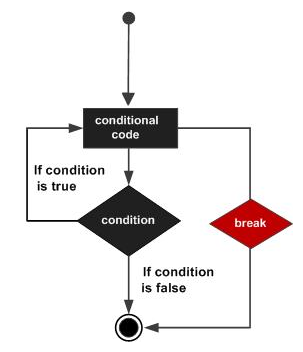Example

`#include <stdio.h> int main () {   /* local variable definition */   int a = 10;   /* while loop execution */   while( a < 20 ) {         printf("value of a: %d", a);      a++;		      if( a > 15) {         /* terminate the loop using break statement */         break;      }		   }    return 0;}`

When the above code is compiled and executed, it produces the following result −

`value of a: 10value of a: 11value of a: 12value of a: 13value of a: 14value of a: 15`

## continue statement

The continue statement in C programming works somewhat like the break statement. Instead of forcing termination, it forces the next iteration of the loop to take place, skipping any code in between.

For the for loop, continue statement causes the conditional test and increment portions of the loop to execute. For the while and do...while loops, continue statement causes the program control to pass to the conditional tests.

## Syntax

The syntax for a continue statement in C is as follows −

`continue;`

## Flow Diagram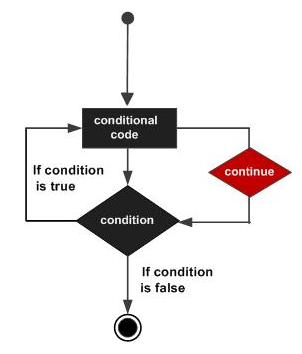## Example

`#include <stdio.h> int main () {   /* local variable definition */   int a = 10;   /* do loop execution */   do {         if( a == 15) {         /* skip the iteration */         a = a + 1;         continue;      }		      printf("value of a: %d", a);      a++;        } while( a < 20 );    return 0;}`

When the above code is compiled and executed, it produces the following result −

`value of a: 10value of a: 11value of a: 12value of a: 13value of a: 14value of a: 16value of a: 17value of a: 18value of a: 19`

## goto statement in

goto statement in C programming provides an unconditional jump from the 'goto' to a labeled statement in the same function.

NOTE − Use of goto statement is highly discouraged in any programming language because it makes difficult to trace the control flow of a program, making the program hard to understand and hard to modify. Any program that uses a goto can be rewritten to avoid them.

## Syntax

The syntax for a goto statement in C is as follows −

`goto label;...label: statement;`

Here label can be any plain text except C keyword and it can be set anywhere in the C program above or below to goto statement.

## Flow Diagram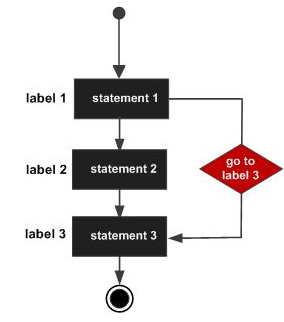## Example

`#include <stdio.h> int main () {   /* local variable definition */   int a = 10;   /* do loop execution */   LOOP:do {         if( a == 15) {         /* skip the iteration */         a = a + 1;         goto LOOP;      }		      printf("value of a: %d", a);      a++;        }while( a < 20 );    return 0;}`

When the above code is compiled and executed, it produces the following result −

`value of a: 10value of a: 11value of a: 12value of a: 13value of a: 14value of a: 16value of a: 17value of a: 18value of a: 19`
Offer running on EduRev: Apply code STAYHOME200 to get INR 200 off on our premium plan EduRev Infinity!

## GATE Computer Science Engineering(CSE) 2022 Mock Test Series

131 docs|167 tests

,

,

,

,

,

,

,

,

,

,

,

,

,

,

,

,

,

,

,

,

,

;